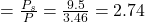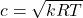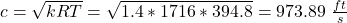The Pitot tube on a supersonic aircraft (see the Video) cruising at an altitude of 35000 ft senses a stagnation pressure of 9.5 psia. If the

Question

The Pitot tube on a supersonic aircraft (see the Video) cruising at an altitude of 35000 ft senses a stagnation pressure of 9.5 psia. If the atmosphere is considered standard, determine (a) the Mach number of the aircraft, (b) the airspeed.

in progress 0
5 months 2021-08-22T11:50:42+00:00 2 Answers 4 views 0

A) Mach number = 1.25

B) Airspeed = 1241.79 m/s

Explanation:

A) From the 1st image attached of the physical properties of air at standard atmospheric pressure; at 35000 ft, by interpolation, we will get a pressure(p) of 3.7 lb/in². From the question, stagnation pressure(Ps) = 9.5 psia or 9.5 lb/in².

Pressure ratio is given as; Ps/P

Thus, pressure ratio = 9.5/3.7 = 2.566

This ratio is tied to mach number and the mach number and from the second image attached, it’s calculated to be approximately 1.25

B) From the first image, from interpolation and at altitude of 35000 ft, Temperature is approximately – 49.2°F.

Now,we need to convert it to degree rankine. Thus temperature (T) = – 49.2 + 460 = 410.8 °R

Gas constant (R) for air when converted to ft.lb/slug °R gives; R=1716 ft.lb/slug °R

Also,for the specific heat ratio, we will use the standard value which is k = 1.4

Now, the speed of sound is given by the equation;

c = √(RkT) = √(1716 x 1.4 x 410.8) = √(986905.92) = 993.43 ft/s

Now for us to calculate the aircraft speed, we will do it from the Mach number equation which is;

Ma = V/c

Where Ma is mach number and V is speed of aircraft and c is speed of sound. In this case, speed of aircraft is equal to the speed of the air relative to the aircraft’s system.

Thus, V =cMa = 993.43 x 1.25 = 1241.79 m/s

(a) mach number is 1.25

(b) airspeed is 1217.36 ft/s

Explanation:

given;

stagnation pressure Ps = 9.5 psia

at 35000 ft, air pressure = 3.46 psia

Part (a) the Mach number of the aircraft

pressure ratio between stagnation pressure and surrounding pressure;At standard specific heat ratio, K value of 1.4, read off mach number from pressure ratio of 2.74

Mach number of air, Ma = 1.25

Part (b) the airspeed

speed of air,Where;

R is gas constant = 1716 Ft.lbf/slug.⁰R

T is air temperature, at 35,000 ft = 394.8 ⁰Rairspeed, V = Ma x c

= 1.25 x 973.89

= 1217.36 ft/s